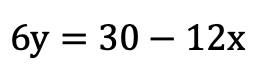// StartMathJax Script window.MathJax = {loader: {load: [ 'input/asciimath', 'ui/lazy', 'output/chtml', 'ui/menu']} }; (function() { var script = document.createElement('script'); script.src = "https://cdn.jsdelivr.net/npm/mathjax@3/es5/startup.js"; script.async = true; document.head.appendChild(script); })(); ---------- (Different files) ---------- // UpdateTypeset Script config = { attributes: true, childList: true, subtree: true }; // Callback function to execute when mutations are observed callback = (mutationList, observer) => { for (mutation of mutationList) { if (mutation.type === 'childList') { console.log('A child node has been added or removed.'); MathJax.typeset(); } else if (mutation.type === 'attributes') { console.log(The \${mutation.attributeName} attribute was modified.); } } }; // Create an observer instance linked to the callback function observer = new MutationObserver(callback); document.onreadystatechange = () => { if (document.readyState === 'complete') { console.log("Loaded fully according to readyState") targetNode = document.getElementById('content-wrapper') console.log(targetNode) // Start observing the target node for configured mutations observer.observe(targetNode, config); } }
top of page
Search

# The Equation for a Straight Line, y = mx + c

Updated: Jul 1, 2022

The formula ​y​ = ​mx​ + ​c​ represents a linear equation that plots a straight line on a graph. The y and x represent the axes, m the gradient and c the y-intercept (where the line crosses the y-axis).

Let’s look at a manipulation to find the equation for a straight-line using the following equation:## Rearrange for y

Subtract 12x from both sides…## Divide all terms by the coefficient of y

This reduces ​y​ to 1The equation gives a straight-line graph with a gradient of -2 and a y-intercept equal to 5.Graph of y = -2x + 5

## Check

Set ​x​ = 0 in your equation (this will give the y-intercept value, c, if your calculation is correct).When x = 0, y = 5 (Setting y = 0 would give you the x-intercept)